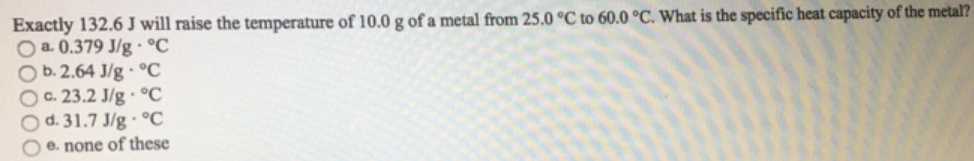Chemistry Calorimetry Solution: Exactly 132.6 J will raise the temperature of 10.0...

# Solution: Exactly 132.6 J will raise the temperature of 10.0 g of a metal from 25.0  C to 60.0 ºC. What is the specific heat capacity of the metal?a. 0.379 J/g -Cb. 2.64 J/g - Cc. 23.2 J/g - Cd. 31.7 J/g -C e. none of these

Problem

Exactly 132.6 J will raise the temperature of 10.0 g of a metal from 25.0  C to 60.0 ºC. What is the specific heat capacity of the metal?

a. 0.379 J/g -C
b. 2.64 J/g - C
c. 23.2 J/g - C
d. 31.7 J/g -C
e. none of theseView Complete Written Solution